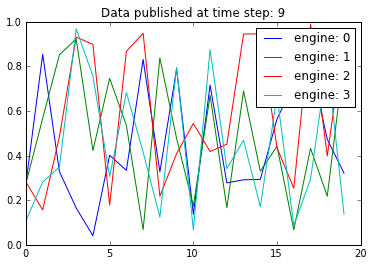# IPython's Data Publication API¶

IPython has an API that allows IPython Engines to publish data back to the Client. This example shows how this API works.

## Setup¶

We begin by enabling pylab mode and creating a Client object to work with an IPython cluster.

In :
%pylab inline

Welcome to pylab, a matplotlib-based Python environment [backend: module://IPython.zmq.pylab.backend_inline].

In :
from IPython.parallel import Client

In :
c = Client()
dv = c[:]
dv.block = False


## Simple publication¶

Here is a simple Python function we are going to run on the Engines. This function uses publish_data to publish a simple Python dictionary when it is run.

In :
def publish_it():
from IPython.zmq.datapub import publish_data
publish_data(dict(a='hi'))


We run the function on the Engines using apply_async and save the returned AsyncResult object:

In :
ar = dv.apply_async(publish_it)


The published data from each engine is then available under the .data attribute of the AsyncResult object.

In :
ar.data

Out:
[{'a': 'hi'}, {'a': 'hi'}, {'a': 'hi'}, {'a': 'hi'}]

Each time publish_data is called, the .data attribute is updated with the most recently published data.

## Simulation loop¶

In many cases, the Engines will be running a simulation loop and we will want to publish data at each time step of the simulation. To show how this works, we create a mock simulation function that iterates over a loop and publishes a NumPy array and loop variable at each time step. By inserting a call to time.sleep(1), we ensure that new data will be published every second.

In :
def simulation_loop():
from IPython.zmq.datapub import publish_data
import time
import numpy as np
for i in range(10):
publish_data(dict(a=np.random.rand(20), i=i))
time.sleep(1)


Again, we run the simulation_loop function in parallel using apply_async and save the returned AsyncResult object.

In :
ar = dv.apply_async(simulation_loop)


New data will be published by the Engines every second. Anytime we access ar.data, we will get the most recently published data.

In :
data = ar.data
for i, d in enumerate(data):
plot(d['a'], label='engine: '+str(i))
title('Data published at time step: ' + str(data['i']))
legend()

Out:
<matplotlib.legend.Legend at 0x10a8ed8d0>